# Law of Conservation of Energy

## Law of Conservation of Energy - Equations and Examples

Conservation refers to the condition where there is no change. So, The variable in an equation, which represents a conserved quantity, is constant over time. Its value remains constant both before and after a particular event.

There are many such conserved quantities in physics. They help in making predictions and making a lot of complicated situations much easier. The three fundamental quantities which are conserved in mechanics are energy, momentum, and angular momentum.

Though energy changes, it still remains conservative in nature. This is because while referring to energy, it is the total energy of a system that is considered. The movement of objects and external factors change one form of energy into another but essentially, energy is not lost anywhere. But, it should be noted that the theory of conservation of energy should only be applied to isolated systems.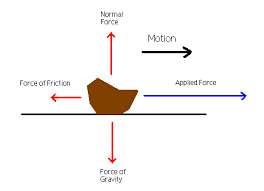This is why a ball rolling across a rough floor is considered not to obey the law of conservation of energy because it is not isolated from the floor. The floor is doing work on the ball through friction, causing changes in the whole system.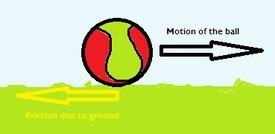However, the ball and floor together form an isolated system and thereby the law of conservation can be applied and it has been proved that it holds true in the combined ball-floor system.

What do we mean by a system?

The system can be considered as the suffix we give to a collection of objects. These objects are modeled by the many standard equations. When we describe the motion of an object using the theory of conservation of energy, then the system is supposed to include the object of interest and all other objects that it interacts with.

But, it should be noted that in practice, some of these interactions have to be ignored and omitted in order to solve problems or make predictions. The system we define has objects and interactions that are of importance to us. We generally concentrate only on them and only they are included. 'Environment' is the term used to call the things we don't include. While this creates an overall inaccuracy, it is considered negligible. One of the qualities that a good physicist should possess is the common sense and ability required to differentiate what should be included in the environment.

For example,

When we consider a problem involving a person bungee jumping from a bridge. At the very minimum, the system should consist of the jumper, bungee, and the Earth. If a more precise calculation should be done, one should include the air, which does work on the jumper via drag, or air resistance. To increase the accuracy, one can include the bridge and its foundation, but the bridge is obviously much heavier than the jumper, hence it can be included in the 'environment'.

What is mechanical energy?

Mechanical energy can be described as the sum of the potential energy and kinetic energy in a system.

Only conservative forces are associated with mechanical energy. This includes forces like gravity and the spring force. Potential energy is associated with this kind of forces. Nonconservative forces like friction and drag do not fall in this category. With conservation forces, if they are added to a system, the energy imperatively retrieved. But on the other hand, recovering energy of the nonconservative forces is very difficult. This is because it often ends up as heat or some other form which mostly ends up outside the system. This can be also described as an energy being lost to the environment.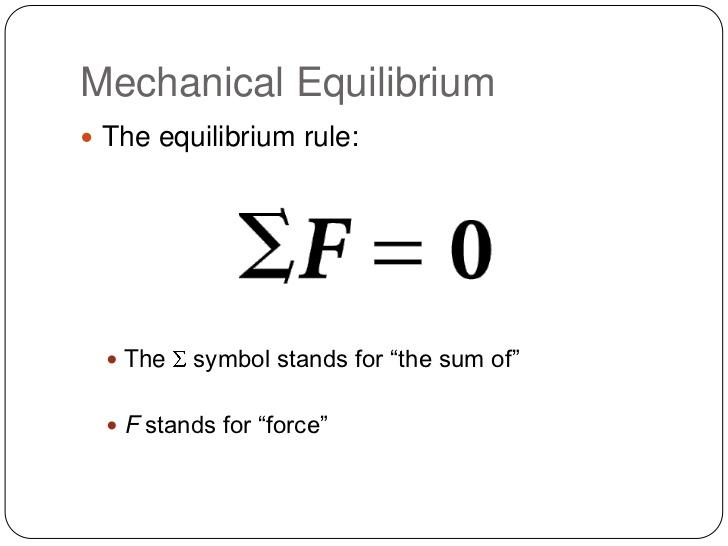In terms of problems and calculations, this means that mechanical energy is much easier to be calculated and used to make predictions. But, it should be remembered that the conservation of mechanical energy only applies when all forces are conservative. Fortunately, in most cases, nonconservative forces can be ignored and included as part of the environment. A good approximation can be made even without adding up the non-conservative forces.

How can conservation of energy explain the movement of objects?

When energy is conserved, it is possible to set up equations since we can equate the sum of the different forms of energy in a system. This allows us to solve the equations for velocity, distance, or any other parameter on which the energy depends. Another advantage of using the theorem of conservation of energy to solve problems is that even if we don’t know all the necessary variables to solve a certain type of problem, it might still be useful in understanding a situation, even in terms of variables.

In a problem which discusses the case of a golfer on the moon striking a golf ball wherein the ball leaves the club at an angle of 45 degrees to the lunar surface traveling at 20 m/s both vertically and horizontally at a total velocity of 28.28 m/s. The question of how high the ball would go can be solved by using the equations associated with the law of conservation of energy and mechanical energy. Such equations are known as kinematic equations.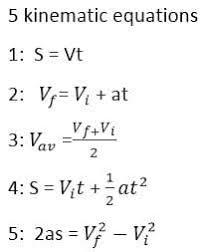Why can perpetual motion machines never work?

The perpetual motion discusses the concept of a machine which continues its motion forever with a condition that there won’t be any reduction in speed. It is the dream of modern science. Though it seems really interesting such a machine cannot really work according to physics we have explored. In fact, even if such a machine were to exist, it wouldn’t be useful to anyone is what has been discovered .apparently, it would have no ability to do work.

According to the principles of mechanics, a system, if it can be fully isolated from the environment and made to subject to only conservative forces, then energy would be conserved and it would run perpetually. The problem we would face is that that in reality, there is no way to completely isolate a system. Also, energy can never be completely conserved within the machine.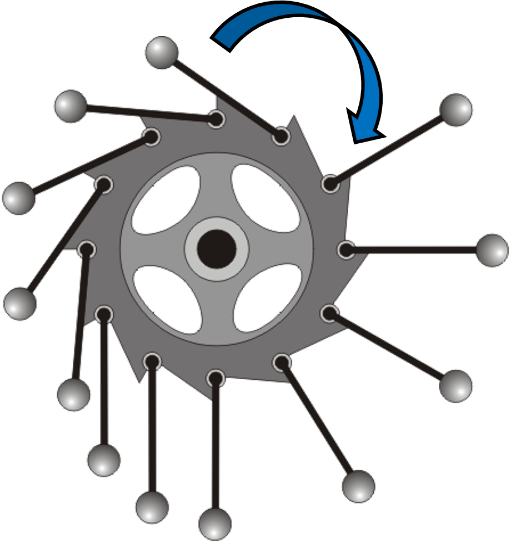Modern science has made extremely low friction flywheels. They rotate in a vacuum in order to store energy. Yet, we have seen that they still lose energy and eventually spin down when unloaded, over a period of years which can be predicted if other factors of the machine and environment are taken into consideration and calculated accordingly. Many physicists and researchers have thought of the earth itself as an extreme example of such a machine. While it rotates on its axis, interactions with the moon, tidal friction, and other celestial bodies, it too is gradually slowing. An unknown but very interesting and terrifying fact is, every couple of years, scientists add a leap second to our record of time to account for the variation in the length of day.